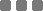## Wednesday, July 24, 2013

### Math: The Proof 1 ≠ 0

This is just for fun. Math is one of my diversified hobbies. Excellent choice of hobby indeed.

I'm gonna find what's wrong with the "proof" on Mudd Math Fun Facts www.math.hmc.edu/funfacts/ffiles/10001.1-8.shtml

Quoted

It looks so COOL at first. BUT, there was a subtle, but actually a giant, flaw in it.

The 'error' occurred in the division part.

Since x = y, if we use (x - y) as the divisor, meaning that we're dividing both sides with 0 (zero).

And also, let's take a look at the the quadratic equation (the dividend) which was being divided,

x² - y² = xy - y²

The thing which isn't acceptable ► when 0 on both sides were divided also by 0.

(x² - y²)/(x - y) = (xy - y²)/(x - y)

Once again, since x = y, hence:

(x - y) = (x² - y²) = (xy - y²) = 0

If we substitute the manipulation with the actual values:

0/0 = 0/0 ? BIG no no
0/0 has undefined or undetermined value.

Meaning that we CAN'T continue the "proofing" steps with dividing both sides like that. It must be stopped there on the subtraction step. Other operation (+, -, exponent, root, logarithmic, integral, etc) besides division can be added, but it will give the same result, that is 0 = 0 (or other same number 1 = 1, 2 = 2, etc).

You can do another kind of different starting manipulation steps for those variables (x and y), and you'll still be using the 0 division to achieve "1 = 0". I'm 100% sure, especially for Algebra manipulation.
There's no proof that 1 = 0 in Mathematics.
Well, there is. It is called magic trick. Not suitable for engineering thing.

Anyway, if there's such thing, then, I don't know, I can transform myself to be a bird or something.
This "special" 0 division "anomaly" happens in Math, Physics, and engineering in general.

So, even though the proving steps were using variables, BUT if we find 0 thingy, we have to be extra careful.

It's like this, when we're gonna drink a cup of coffee, but it actually isn't. The foggy-looks-like-drinkable stuff inside the cup has the same color as coffee, turns out, it's a cup of liquid asphalt. That's why most of us have nose.

Pretty weird?
Yup.

In conclusion, the manipulated "proof" above is a fun time to test your keenness in basic Algebra.